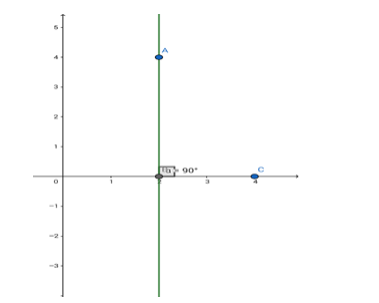# Find the equation of the line passing through the point (2, 4) and`
Question:

Find the equation of the line passing through the point (2, 4) and perpendicular to the x - axis.

Solution:Given: The line is perpendicular to $x$-axis and passes through $(2,4)$

The equation of the line perpendicular to the $x$-axis $(y=0)$ can be represented as $x=$ c, where $c$ is a real constant.

Now, this line passes through (2,4).

∴ c = 2

The required equation is x = 2